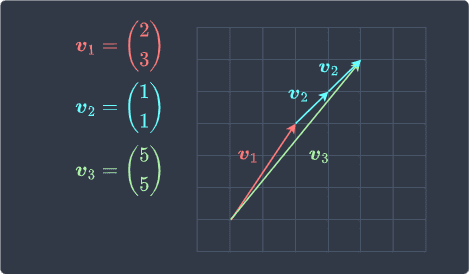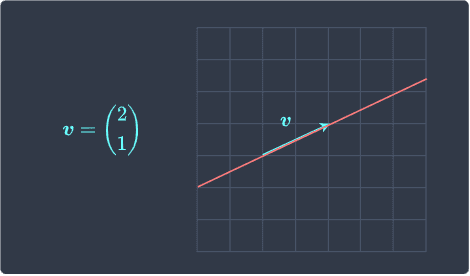search
Search
Unlock 100+ guides
search toc
close
account_circle
Profile
exit_to_app
Sign out
search
keyboard_voice
close
Searching Tips
Search for a recipe:
"Creating a table in MySQL"
Search for an API documentation: "@append"
Search for code: "!dataframe"
Apply a tag filter: "#python"
Useful Shortcuts
/ to open search panel
Esc to close search panel
to navigate between search results
d to clear all current filters
Enter to expand content previewDoc SearchCode Search BetaSORRY NOTHING FOUND!
mic
Start speaking...Voice search is only supported in Safari and Chrome.
Shrink
Navigate to

# Guide on Spanning Set of a Vector Space

schedule Aug 11, 2023
Last updated
local_offer
Linear Algebra
Tags
mode_heat
Master the mathematics behind data science with 100+ top-tier guides
Start your free 7-days trial now!
Definition.

# Spanning set of a vector space

Let $S=\{\boldsymbol{v}_1,\boldsymbol{v}_2,\cdots,\boldsymbol{v}_n\}$ be a subset of a vector space $V$. The set $S$ is called a spanning set of $V$ if every vector in $V$ can be written as a linear combinationlink of vectors in $S$. In such a case, we say that $S$ spans $V$.

Example.

# Checking if a vector is contained in a spanning set

Consider the following three vectors:

$$\boldsymbol{v}_1 = \begin{pmatrix} 2\\3 \end{pmatrix},\;\;\;\;\; \boldsymbol{v}_2=\begin{pmatrix} 1\\1 \end{pmatrix},\;\;\;\;\; \boldsymbol{v}_3=\begin{pmatrix} 4\\5 \end{pmatrix}$$

Show that $\boldsymbol{v}_3$ is contained in the vector space spanned by $\boldsymbol{v}_1$ and $\boldsymbol{v}_2$.

Solution. The spanning set of $\boldsymbol{v}_1$ and $\boldsymbol{v}_2$ holds all vectors that can be expressed as a linear combination of $\boldsymbol{v}_1$ and $\boldsymbol{v}_2$, that is:

$$\mathrm{span}(\boldsymbol{v}_1,\boldsymbol{v}_2)= \{c_1\boldsymbol{v}_1+c_2\boldsymbol{v}_2\;|\;c_1,c_2\in\mathbb{R}\}$$

To show that $\boldsymbol{v}_3$ is included in this spanning set, we must find scalars $c_1$ and $c_2$ such that:

$$\begin{equation}\label{eq:QSK03HjQ0KwAVv4bM5a} c_1\boldsymbol{v}_1+ c_2\boldsymbol{v}_2= \boldsymbol{v}_3\;\;\;\;\; \Longleftrightarrow\;\;\;\;\; c_1\begin{pmatrix} 2\\3\\ \end{pmatrix}+ c_2\begin{pmatrix} 1\\1\\ \end{pmatrix} = \begin{pmatrix} 4\\5\\ \end{pmatrix} \end{equation}$$

Rewriting the left-hand side as a matrix-vector product:

$$\begin{pmatrix} 2&1\\3&1\\ \end{pmatrix} \begin{pmatrix} c_1\\c_2\\ \end{pmatrix} = \begin{pmatrix} 4\\5\\ \end{pmatrix}$$

Let's use Gaussian elimination to solve for $c_1$ and $c_2$. We row-reduce the augmented matrix to obtain the reduced row echelon form:

$$\begin{pmatrix} 2&1&\color{blue}4\\3&1&\color{blue}5\\ \end{pmatrix}\sim \begin{pmatrix} 2&1&\color{blue}4\\-1&0&\color{blue}-1\\ \end{pmatrix}\sim \begin{pmatrix} 2&1&\color{blue}4\\1&0&\color{blue}1\\ \end{pmatrix}\sim \begin{pmatrix} 0&1&\color{blue}2\\1&0&\color{blue}1\\ \end{pmatrix} \sim \begin{pmatrix} 1&0&\color{blue}1\\0&1&\color{blue}2\\ \end{pmatrix}$$

We have the result that $c_1=1$ and $c_2=2$. Therefore, \eqref{eq:QSK03HjQ0KwAVv4bM5a} becomes:

$$\boldsymbol{v}_1+ 2\boldsymbol{v}_2= \boldsymbol{v}_3\;\;\;\;\; \Longleftrightarrow\;\;\;\;\; \begin{pmatrix} 2\\3\\ \end{pmatrix}+ 2\begin{pmatrix} 1\\1\\ \end{pmatrix} = \begin{pmatrix} 4\\5\\ \end{pmatrix}$$

Because we have managed to express $\boldsymbol{v}_3$ as a linear combination of $\boldsymbol{v}_1$ and $\boldsymbol{v}_2$, we conclude that $\boldsymbol{v}_3$ is included in the spanning set $\mathrm{span}(\boldsymbol{v}_1,\boldsymbol{v}_2)$.

Visually, $\boldsymbol{v}_3$ can be constructed by adding one $\boldsymbol{v}_1$ and two $\boldsymbol{v}_2$ like so:Example.

# Showing that vectors span a vector space

Consider the following vectors:

$$\boldsymbol{v}_1 = \begin{pmatrix} 2\\1 \end{pmatrix},\;\;\;\;\; \boldsymbol{v}_2=\begin{pmatrix} 1\\3 \end{pmatrix}$$

Show that these vectors span the vector space $\mathbb{R}^2$.

Solution. To show that these vectors span the vector space $\mathbb{R}^2$, we need to show that any vector in $\mathbb{R}^2$ can be expressed as a linear combination of $\boldsymbol{v}_1$ and $\boldsymbol{v}_2$. Let $\boldsymbol{b}$ be any vector in $\mathbb{R}^2$ defined like so:

$$\boldsymbol{b}= \begin{pmatrix} b_1\\b_2 \end{pmatrix}$$

We need to show that there exist scalar constants $c_1$ and $c_2$ such that:

$$\boldsymbol{b}= c_1\boldsymbol{v}_1+c_2\boldsymbol{v}_2 \;\;\;\;\;\Longleftrightarrow\;\;\;\;\; \begin{pmatrix} b_1\\b_2 \end{pmatrix}= c_1\begin{pmatrix} 2\\3 \end{pmatrix}+ c_2\begin{pmatrix} 1\\4 \end{pmatrix}$$

By theorem, the right-hand side can be expressed as a matrix-vector product:

$$\begin{pmatrix} b_1\\b_2 \end{pmatrix}= \begin{pmatrix} 2&3\\ 1&4\\ \end{pmatrix} \begin{pmatrix} c_1\\c_2 \end{pmatrix}$$

We know from theoremlink that we can check for the existence of a solution using the row echelon form of the coefficient matrix:

$$\begin{pmatrix} 2&3\\1&4\\ \end{pmatrix}\sim \begin{pmatrix} 1&3\\0&-5\\ \end{pmatrix}\sim \begin{pmatrix} 1&3\\0&1\\ \end{pmatrix}\sim \begin{pmatrix} 1&0\\0&1\\ \end{pmatrix}$$

Again by theoremlink, this means that $c_1$ and $c_2$ exist. Since any vector $\boldsymbol{b}$ in $\mathbb{R}^2$ can be expressed using a linear combination of $\boldsymbol{v}_1$ and $\boldsymbol{v}_2$, we conclude that the two vectors indeed span the vector space $\mathbb{R}^2$.

Example.

# Showing that vectors do not span a vector space

Consider the following two vectors:

$$\boldsymbol{v}_1 = \begin{pmatrix} 1\\2 \end{pmatrix},\;\;\;\;\; \boldsymbol{v}_2=\begin{pmatrix} 2\\4 \end{pmatrix}$$

Show that the vectors do not span $\mathbb{R}^2$.

Solution. Let $\boldsymbol{b}$ be any vector in $\mathbb{R}^2$. We check if there exists scalars $c_1$ and $c_2$ that can generate $\boldsymbol{b}$ like so:

$$\boldsymbol{b}= c_1\boldsymbol{v}_1+c_2\boldsymbol{v}_2 \;\;\;\;\;\Longleftrightarrow\;\;\;\;\; \begin{pmatrix} b_1\\b_2 \end{pmatrix}= \begin{pmatrix} 1&2\\ 2&4\\ \end{pmatrix} \begin{pmatrix} c_1\\c_2 \end{pmatrix}$$

The augmented matrix is:

$$\begin{pmatrix} 1&2&b_1\\2&4&b_2\\ \end{pmatrix}$$

For simplicity, we can ignore the right-most column and just acknowledge that its values will change as we perform elementary row operations:

$$\begin{pmatrix} 1&2&\color{blue}*\\2&4&\color{blue}*\\ \end{pmatrix}\sim \begin{pmatrix} 1&2&\color{blue}*\\1&2&\color{blue}*\\ \end{pmatrix}\sim \begin{pmatrix} 1&2&\color{blue}*\\0&0&\color{blue}*\\ \end{pmatrix}$$

Here, $\color{blue}*$ is some scalar value. If $\color{blue}*$ is non-zero, then a solution does not exist. Therefore, not every vector in $\mathbb{R}^2$ can be expressed as a linear combination of $\boldsymbol{v}_1$ and $\boldsymbol{v}_2$. In other words, the vectors do not span $\mathbb{R}^2$.

Example.

# Spanning set of a single vector

Consider the following vector:

$$\boldsymbol{v}= \begin{pmatrix} 2\\1 \end{pmatrix}$$

Find the vector space spanned by $\boldsymbol{v}$.

Solution. The spanning set of $\boldsymbol{v}$ is the set of all the linear combinations of $\boldsymbol{v}$. The linear combination of a single vector $\boldsymbol{v}$ is just the scalar multiple of $\boldsymbol{v}$, that is:

$$\mathrm{span}(\boldsymbol{v})=\{cv\;|\;c\in\mathbb{R}\}$$

We know that multiplying vectors by scalars results in either stretching/shrinking the vector without affecting its direction. Therefore, the spanning set of $\boldsymbol{v}$ is the set of all vectors that lie on the red line traced out by $\boldsymbol{v}$ like so:Examples of vectors that are contained in this spanning set are:

$$\begin{pmatrix} 4\\2 \end{pmatrix},\;\;\;\;\; \begin{pmatrix} -2\\-1 \end{pmatrix},\;\;\;\;\; \begin{pmatrix} 8\\4 \end{pmatrix}$$

Keep in mind that $\boldsymbol{v}$ does not span $\mathbb{R}^2$ because there exist many vectors in $\mathbb{R}^2$ that are not contained in the spanning set - for example:

$$\begin{pmatrix} 2\\2 \end{pmatrix},\;\;\;\;\; \begin{pmatrix} 2\\-1 \end{pmatrix},\;\;\;\;\; \begin{pmatrix} 4\\1 \end{pmatrix}$$
thumb_up
thumb_down
Comment
Citation
Ask a question or leave a feedback...
thumb_up
0
thumb_down
0
chat_bubble_outline
0
settings
Enjoy our search
Hit / to insta-search docs and recipes!